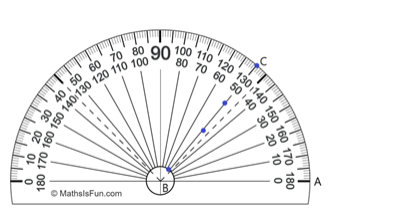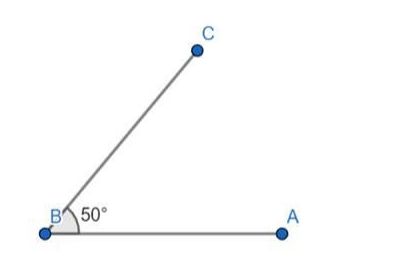# Using a protractor, draw each of the following angles.

Question:

Using a protractor, draw each of the following angles.

$-310^{\circ}$

Solution:

The given angle is negative

Adding or subtracting $360^{\circ}$ from a particular angle does'nt changes its position.

Therefore, Angle can also be written as $=-310^{\circ}+360^{\circ}=50^{\circ}$- Draw a straight line AB.

- Place a dot at B. This dot represents the vertex of the angle.

- Place the centre of the protractor at B and the baseline of the protractor along the arm BA.

- Find $50^{\circ}$ on the scale and mark a small dot at the edge of the protractor.

- Join the vertex $B$ to the small dot with a ruler to form the second arm, $B C$, of the angle.

- Mark the angle with a small arc as shown below.